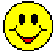The Pulsoid TheoryA Light vector, heuristically described in two dimensions, has coordinate axes, which are diameters of an ellipse, one axis is linear, the other cyclical.  The coordinate axes express time; the vector expresses direction.The two-dimensional heuristic vector is actually a complex tensor of a dynamic ellipsoid, which is referred to as a Pulsoid.Pulsoids exist and expand nonlinearly, cyclically, and quaquaversally dependent on the unit values and ratios of their constituent solitons.Thus, the internal structure of Light . . . and all other phenomena.And, thus, at once, is explained the confusion concerning both the definition of time, which is a dimension that is, simultaneously, linear and cyclical; and, the enigmatic manifestation of Light as either a particle or a wave.It is the Pulsoid and its relationship to a definition of Infinity as both the Singularity and a duality that is the crux of the Equilibrium Theory of Reality, which is commonly referred to as the Pulsoid Theory.